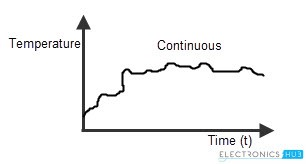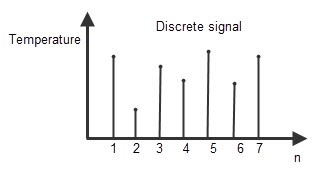# Electronic System

An Electronic System is a physical interconnection of components, or parts, that gathers various amounts of information together. It does this with the aid of input devices such as sensors, that respond in some way to this information and then uses electrical energy in the form of an output action to control a physical process or perform some type of mathematical operation on the signal. But electronic control systems can also be regarded as a process that transforms one signal into another so as to give the desired system response. Then we can say that a simple electronic system consists of an input, a process, and an output with the input variable to the system and the output variable from the system both being signals. There are many ways to represent a system, for example: mathematically, descriptively, pictorially or schematically. Electronic systems are generally represented schematically as a series of interconnected blocks and signals with each block having its own set of inputs and outputs. As a result, even the most complex of electronic control systems can be represented by a combination of simple blocks, with each block containing or representing an individual component or complete sub-system. The representing of an electronic system or process control system as a number of interconnected blocks or boxes is known commonly as “block-diagram representation”.

Block Diagram Representation of a Simple Electronic System

Types of Electronic Systems
As we already explained earlier, every control system will have its own input and output. The input signal may be of continuous and discrete. Based on the input provided to the system, the control systems are classified into 2 types. They are

• Continuous system: Continuous system is that in which the input signals are defined as the analog signals. The analog signals are continuous in nature, so they are called as “continuous time signals”. The magnitude of the continuous signal will vary with the time period T. As the input is Analogues in nature, the continuous electronic systems produce the analogous output. This is the reason they are notified as “Pure analog systems”. The values of the continuous electronic system varies only with time.source: Electronics Hub

Example for continuous system
The process of measuring the temperature of a room is an example of continuous system. As the temperature is measured in a range of time duration, like Monday to Saturday or temperature levels from cold to hot etc, the temperature monitoring system is analog in nature or it is said to be an example of continuous electronic system. Other examples of the continuous electronic systems are current and voltage signals, pressure, velocity and temperature signal etc.

• Discrete system: The discrete system is defined as the system having the sequence of discrete time values as its input. The values obtained at particular intervals of time are called “Discrete values”. As the input of discrete system is the sequence of instinctual values, the output will be in the form of sequence of numbers. So these discrete systems are also known as “Digital systems”. Generally the output of digital system will be a binary number sequence, such as the collection of 0’s and 1’s. One important thing about the discrete system is that, the discrete values defined at particular time intervals must be spaced equally. For example, if the time periods are t1, t2, t3, t4 and t5, then t1 – t2 = t2 – t3 = t3 – t4 = t4 – t5. The values of discrete system don’t vary over the time period, but changes at particular instances of time.source: Electronics Hub

Example for Discrete system
The temperature measuring (monitoring) system which measures the temperature of room only at particular intervals of times, such as particular days like on Monday, on Tuesday or in particular time intervals like 1pm, at 2pm, at 3pm etc is the example of discrete time electronic system.

Representing continuous system as a set of discrete intervals We can represent the continuous electronic system into discrete electronic system, by changing the domain of the system. This means, a continuous system with input and output signals as X (t) and Y (t) can be represented as discrete electronic system with input and output as X (n) and Y (t), where X (t) and Y (t) represents the time domain and X (n) and Y (n) represents the frequency domain

Electric Vs. Electronic System
Electronic and electric devices are not synonymous. An electric device is one that directly uses electrical energy to perform a task. The motion and energy of electrons within the conducting wires and interconnected components is primary to the operation of the device. On the other hand, an electronic device is defined as a device that operates on electrical energy in order to fulfill a task. The information carried by the electrons is operated on and used by the device to perform its function. Typically, electronic devices also have an integrated electrical section, the power supply, that appropriates voltage and current to the electronic components of the rest of the device. In this case, we would usually denote the product as electronic according to its principal purpose, although the distinction is blurred when looking at large, complex systems.

For example, early cars were entirely mechanical devices, but became electrical machines upon the advent of the internal combustion engine, which required a spark plug and a magneto. Later, once digital clocks and dashboard notification systems became commonplace, cars included an integrated package of electronics, such as on-board computers to help control the car, provide information to the driver, and serve auxiliary functions. Today, cars involve a mix of electrical and electronic systems that are seamlessly integrated. Referring to a car in its entirety as electronic or electrical betrays the fact that cars are complex machines with many subsystems that cannot be so easily categorized (note that the term "electric car" falls outside of this type of discussion because it refers to a car principally powered by electricity rather than a gasoline-based engine).